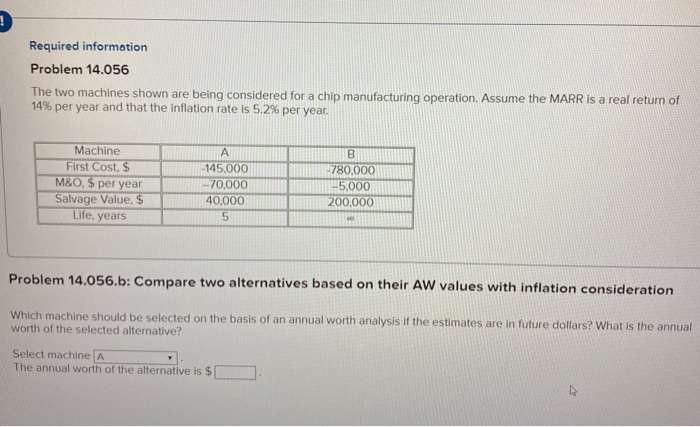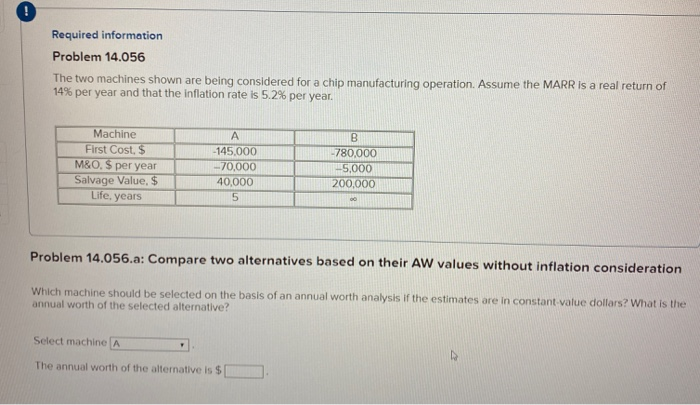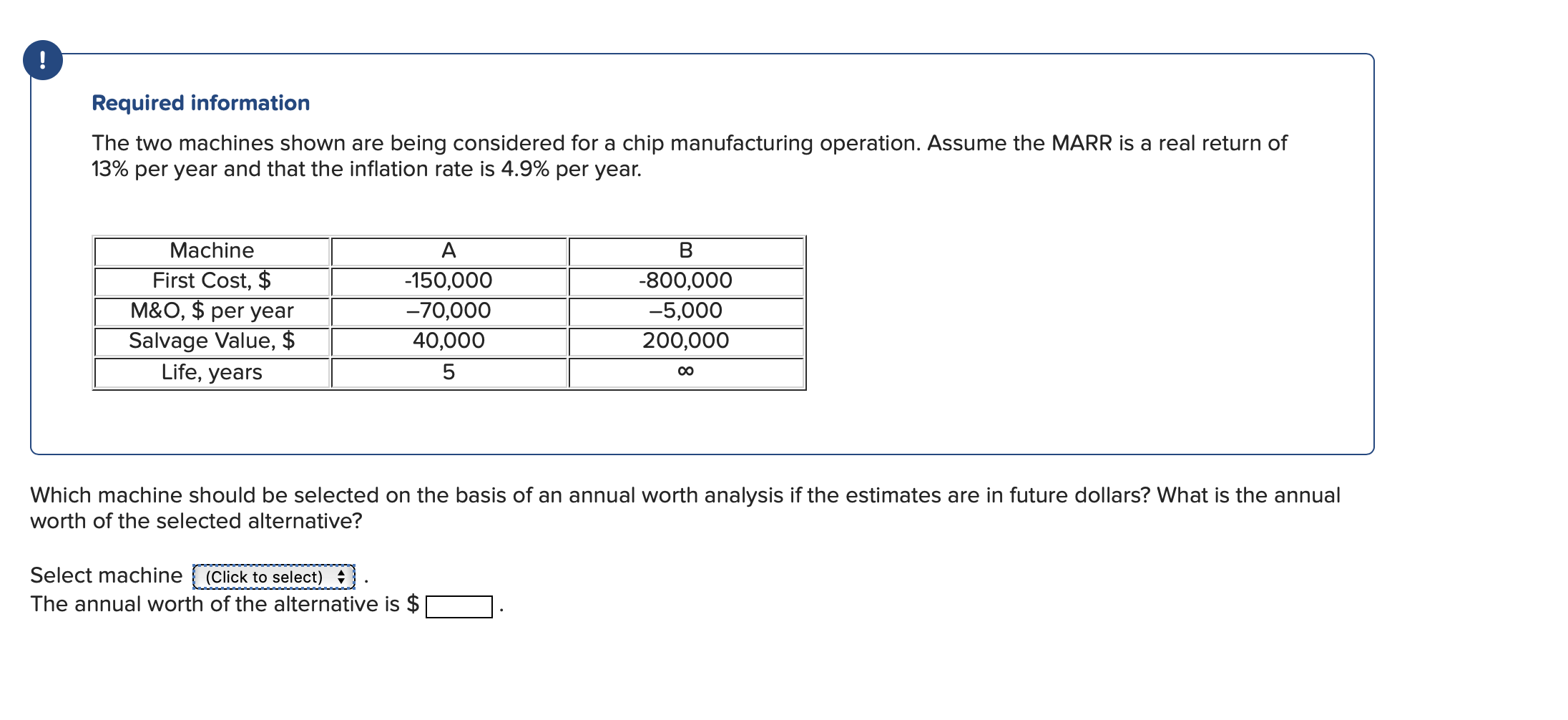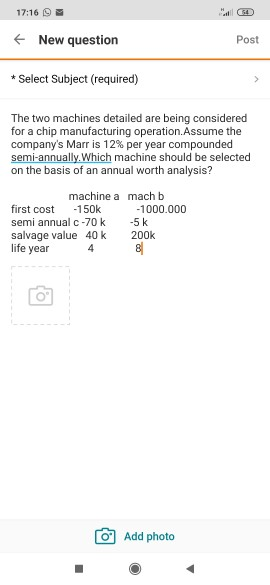Question

# Q8

For th below two machines and based on CC analysis which machine we should select? MARR=10%

 Machine A Machine B First cost, \$ 21,439 124,845 Annual cost, \$/year 13,160 7,237 Salvage value, \$ 5,419 - Life, years 3 infinite

B- the CC for machine B=

SOLUTION :

r = MARR = 10% = 0.1

=> (1 + r) = 1.1

PV of costs of Machine A :

= 21439 + 13160/ 1.1 + 13160/1.1^2 + 13160/1.1^3 - 5419/1.1^3

= 50094.60 (\$)

PV of costs of Machine B :

= 124845 + 7237 / 0.1

= 197215 (\$)

Therefore, as per CC, Machine A has lower cost. So Machine A should be selected.

#### Earn Coins

Coins can be redeemed for fabulous gifts.

Similar Homework Help Questions
• ### QUESTION 7

or th below two machines and based on CC analysis which machine we should select? MARR=10%.Machine AMachine BFirst cost, \$              24,311          100,000Annual cost, \$/year                 13,553               7,000Salvage value, \$                 6,323 - Life, years 3infiniteAnswer the below question:A- the CC for machine A=

• ### For the below ME alternatives, which machine should be selected based on the AW analysis. MARR=10%

QUESTION 3For the below ME alternatives, which machine should be selected based on the AW analysis. MARR=10%Machine AMachine BMachine CFirst cost, \$26,5383000010000Annual cost, \$/year8,0606,0004,000Salvage value, \$4,0005,0001,000Life, years362Answer the below questions:A- AW for machine A=QUESTION 4For the below ME alternatives, which machine should be selected based on the AW analysis. MARR=10%Machine AMachine BMachine CFirst cost, \$1500021,66710000Annual cost, \$/year8,8706,0004,000Salvage value, \$4,0005,0001,000Life, years362Answer the below questions:B- AW for machine B=

• ### Required information Problem 14.056 The two machines shown are being considered for a chip manufacturing operation....Required information Problem 14.056 The two machines shown are being considered for a chip manufacturing operation. Assume the MARR is a real return of 14% per year and that the inflation rate is 5.2% per year. -780.000 Machine First Cost. \$ M&O. \$ per year Salvage Value, \$ Life, years -145,000 - 70.000 40,000 -5,000 200,000 Problem 14.056.b: Compare two alternatives based on their AW values with inflation consideration Which machine should be selected on the basis of an annual...

• ### Required information Problem 14.056 The two machines shown are being considered for a chip manufacturing operation....Required information Problem 14.056 The two machines shown are being considered for a chip manufacturing operation. Assume the MARR is a real return of 14% per year and that the inflation rate is 5.2% per year. 0.000 Machine First Cost, \$ M&0. \$ per year Salvage Value, \$ Life, years -145.000 -70.000 40,000 5.000 00.000 Problem 14.056.a: Compare two alternatives based on their AW values without inflation consideration Which machine should be selected on the basis of an annual worth...

• ### Required information The two machines shown are being considered for a chip manufacturing operation. Assume the...Required information The two machines shown are being considered for a chip manufacturing operation. Assume the MARR is a real return of 13% per year and that the inflation rate is 4.9% per year. Machine First Cost, \$ M&0, \$ per year Salvage Value, \$ Life, years А -150,000 -70,000 40,000 B -800,000 -5,000 200,000 00 Which machine should be selected on the basis of an annual worth analysis if the estimates are in future dollars? What is the annual...

• ### question 3

For the below ME alternatives , which machine should be selected based on the PW analysis. MARR=10%Machine AMachine BMachine CFirst cost, \$              24,425            30000          10000Annual cost, \$/year                 8,323               6,000            4,000Salvage value, \$                 4,000               5,000            1,000Life, years 362Answer the below questions :A- PW for machine A=

• ### QUESTION 3

For the below ME alternatives , which machine should be selected based on the PW analysis. MARR=10%Machine AMachine BMachine CFirst cost, \$              27,272            30000          10000Annual cost, \$/year                 9,401               6,000            4,000Salvage value, \$                 4,000               5,000            1,000Life, years 362Answer the below questions :A- PW for machine A=

• ### immediately please immediately 17:16 al ( + New question Post * Select Subject (required) The two...immediately please immediately 17:16 al ( + New question Post * Select Subject (required) The two machines detailed are being considered for a chip manufacturing operation. Assume the company's Marr is 12% per year compounded semi-annually. Which machine should be selected on the basis of an annual worth analysis? machine a mach b first cost -150k -1000.000 semi annual C-70k -5 k salvage value 40k 200k 81 life year 4 10 Add photo

• ### QUESTION 4

or the below ME alternatives , which machine should be selected based on the PW analysis. MARR=10%Machine AMachine BMachine CFirst cost, \$              15000            27,898          10000Annual cost, \$/year                 8,415               6,000            4,000Salvage value, \$                 4,000               5,000            1,000Life, years 362Answer the below questions :B- PW for machine B=

• ### For the below ME alternatives , which machine should be selected based on the PW analysis. MARR=10%.

For the below ME alternatives, which machine should be selected based on the PW analysis. MARR=10%.Machine AMachine BMachine CFirst cost, \$              15000            30000          10,360Annual cost, \$/year                 8,320               6,000            4,000Salvage value, \$                 4,000               5,000            1,000Life, years 362Answer the below questions :C- PW for machine C =